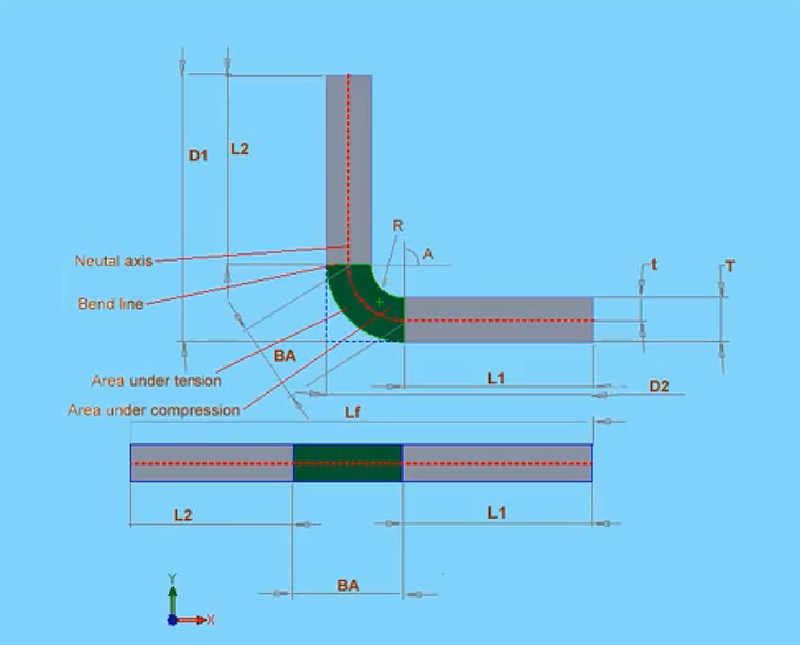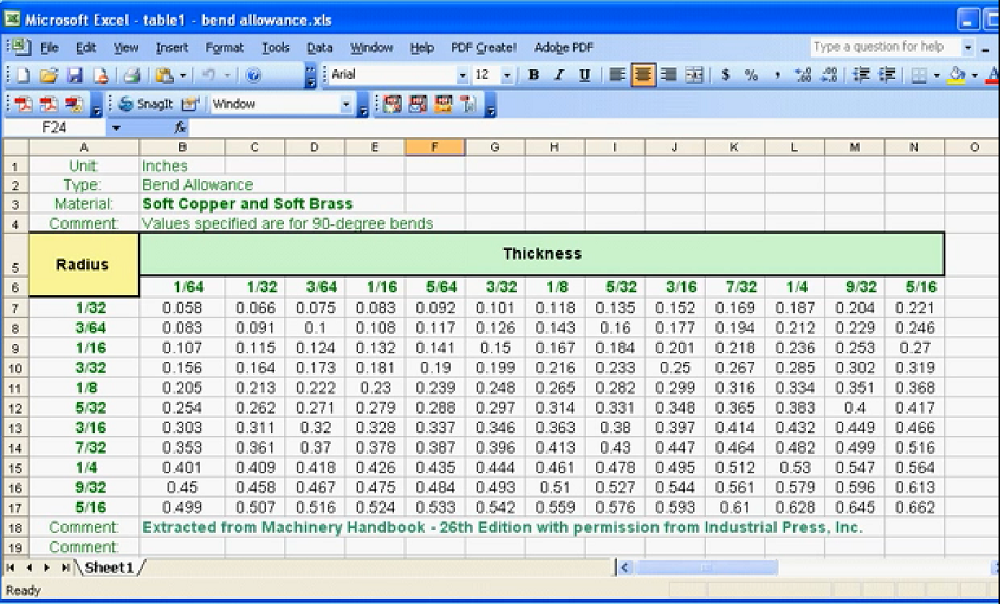# Bend Allowance: Sheet Metal Part Design (Solidworks Tutorial)

Today we will learn the method to calculate bend allowance.

You can directly use our bend allowance calculator to calculate the bending allowance. Besides, the fabrication calculator can also help you calculate K-factor, Y-factor, bend allowance, bend deduction, etc.

You may be wondering what exactly is bend allowance if you’ve never worked with sheet metal before.

Well, when a sheet is bent in the press brake, the part of the sheet close to and in contact with the punch elongate to compensate for the given bend.

If you compare the length of this part, before and after the bending, you’re going to find that they’re different.

As engineers, if you don’t compensate for this variation, the final product won’t have accurate dimensions.

Of course, this is more critical for parts where you’ve got to maintain a tighter allowance or precision.

In this post, I do cover some of the basic problems and principles you have to deal with regularly when working with sheet metal.

Before we get rolling, I want to comment on something, there is not really a scientific method formula for determining a truly exact calculation of the bend allowance, and that’s because there are so many factors at play during the production of your sheet metal part.

For example, actual material thickness and infinite variety of tooling conditions, forming methods, on and on and on.

There are many variables here, and in reality, many methods are used to calculate the bend allowance.

Trial and error is probably the most popular one, bend tables are another popular method.

Bend tables are usually available for metal suppliers, manufactures as well as in engineering textbooks.

Some companies develop their own bending tables based upon their own standard formulas.

Let’s get back to Solidworks.

How exactly the Solidworks calculate bend allowance?

Solidworks actually uses two methods: bend allowance and bend deduction.

## Bending Allowance

I’m going to explain what these methods are and show you how they’re using in Solidworks.The bend allowance method is based on the formula that I’ve got appear in my diagram.

• Lf = L1 + L2 + BA
• BA = Bend Allowance

The total length of the flatten sheet is equal to the sum of L1 that’s the first length, and L2 plus the bend allowance.

The bend allowance region is shown in green in my diagram, this is a region wherein theory all deformation occurs during the bending process.

Generally, the bend allowance is going to be different for each combination of material type, material thickness, bend radius, bend angle and different machining processes, types, speeds and so on.

Truly the list of potential variables is very extensive.

The value of the bend allowance that comes from sheet metal suppliers and manufactures, as well as engineering textbooks, are provided in bend tables and bend table looks pretty much like the following excel spreadsheet.The bend table approach is probably the most accurate approach for calculating bend allowance.

You can input your data manually into a matrix of the bend angle and bend radius.

If you not sure of the bend allowance value you can run some tests.

You need a piece of the exact same sheet metal you’re using to manufacture your part, and then you bend it using the exact processes that you’ll be using during your machining.

Just take some measurements before and after the bending and then based on the same information you can adjust the bend allowance needed.

## Bend Deduction

Another method that Solidworks uses is the bend deduction method.

The formula is as follows:

• Lf = D1 + D2 – BD
• BD = Bend Deduction

The flatten length of the parts that are Lf, equals D1 plus D2, minus the bend deduction.

As with bend allowance, bend deduction comes from the same sources, tables and manual testing.

As you can see, it’s easy to imagine how these values are related to each other based on what these formulas indicate.

• L1 + L2 + BA = D1 + D2 – BD

## K-factor

Another method for calculating bend allowance uses the k-factor.

Well, K is the neutral axis offset.

The general principle of this formula goes like this:

The neutral axis is in my diagram here and red. They don’t change during the bending process.

During the bending process, the material inside the neutral axis will compress, and material outside the neutral axis will stretch.

The neutral axis would be closer to the inside bend.

The inside bend is indicated in blue in the diagram.

The more the part bends, the closer the neutral axis will lie to the inside of the part.

Bend allowance calculation formula with K-factor:

BA = 2πA(R+KT)/360

• π=3.14
• A=Angle (degrees)
• K=Neutral Axis Offset (K-factor) t/T
• T=Thickness of Material
• BA=Length of Bend Allowance

The k-factor equals t or the offset distance to the neutral axis. We divide that by big T, that’s the thickness of the material.

In this formula here the bend allowance equals 2 times pi, multiplied by A that’s the angle, multiplied by the sum of R the bend radius, plus the k-factor, multiply by T the thickness of the material, and then you divide all of this by 360.

In theory, the k-factor can be anywhere between 0 and 1, but for practical purposes, it’s more like 0.25 –  0.5.

• K-Factor = 0 – 1 (in theory)
• K-Factor = 0.25 – 0.5 (practical)

For example, you’ll find that hard materials like steel have got a higher k-factors such as 0.5, soft materials like copper or brass are going to have a lower k-factor closer to 0.5.

And don’t worry that’s the last formula would be walking through in this lesson, it might seem a little bit confusing now, but once you practice a little bit it’ll become second nature.One last point here let me take a look at the example, there is hem on this part, it’s got a k-factor or something like 0.3.

On the other hand, a soft bend or for example the gradual bend that I’ve got on the other side of this part is got a higher k-factor of about 0.5, and this concludes our lesson on bend allowance.

Maybe you ...

## Need a Quote? Have Questions?

### 1 thought on “Bend Allowance: Sheet Metal Part Design (Solidworks Tutorial)”

1. Hello, I’ve been designing parts and following your bend allowance calculator but I don’t think I am using it correctly. I use a program called Shapr3D.

For example, when I design a part such as a 90 degree bend in a 1/4” thick L-bracket, with 4” long flanges. I input the interior radius as 1/4”, angle at 90 degrees, and the exterior radius is calculated for me at .5537”.

The problem I run into is when I sent this part out as a .step file for flattening, I’ve been told that the other CAD programs like fusion or solid works, the flatten process errors and there lies and issue with the radii.

Is this something you can assist with?

Hello friend
Before you leave...
Have any questions or price requests? Don't miss the last chance for our experts to reply your inquiry.
Contact Expert Team
Your message will be replied within 12 hours.Scroll to Top